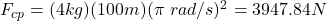Question

Calculate the centripetal force on the end of a 100 m (radius) massless cable that is rotating at 0.5 rev/s. Assume the mass on the end of the cable is 4 kg.

1.thanhcong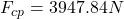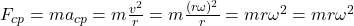Since one revolution is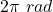, 0.5 revolutions are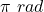, so we have: